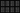Magic Squares

15 March 2009 — written by Prakash PrasadA Magic Square is an arrangement of the distinct positive integers $1, 2, …, {n}^2$ such that the sum of all the rows, columns and main diagonals is always the same number. This Number that all Rows, Columns and main Diagonals add up to is known as the Magic Constant.

The Magic Constant for an $N \times N$ Magic Square is represented by:

$\frac{N ({N}^2 + 1)}{2}$

The logic behind this constant is very trivial and can be deduced by anyone who knows the following formula:

$\frac{N (N + 1)}{2}$

Yes. It is the sum of the first N Natural Numbers. In a Magic Square, the numbers that can be used are $1, 2, …, {n}^2$. Since we have the first ${N}^2$ Natural Numbers, the sum of the whole magic square is:

$T = \frac{{N}^2 ({N}^2 + 1)}{2}$

Also, the sum of each row (or column) is going to be same. Let it be $X$. Then the total sum of the Square will be $N \times X$. This has to be equal to the total sum calculated above. So, the sum of numbers in each row (and hence, each column) is:

$M = \frac{T}{N}$

For a $3 \times 3$ grid, there are $8$ possible arrangements that qualify as Magic Squares.

Note: The Magic Squares in this post have been generated by using a Brute Force Algorithm which goes through all possible permutations (about $9! = 362880$; the next one for $4$ being $16! = 20922789888000$).